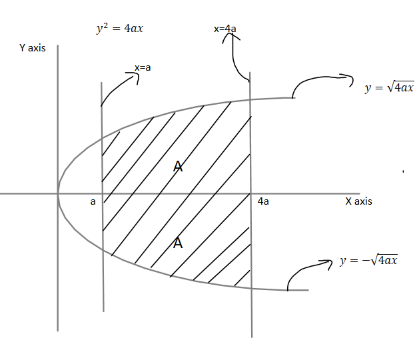QuestionAnswers

# Area inside the parabola ${y^2} = 4ax$ between the lines $x = a\,\& \,x = 4a$ is equal to$a) \,4{a^2} \\ b) \,8{a^2} \\ c) \,\dfrac{{28}}{3}{a^2} \\ d) \,none\,of\,these \\$

Hint: Area inside a parabola can be found using definite integration from $x = a\,to\,x = 4a$. As integration always gives area under the curve being represented. We have to integrate the function $y = f(x)$here $y = \sqrt {4ax}$
Area $= \int\limits_a^{4a} {f(x)dx}$

Here, $y = \sqrt {4ax}$
Area $= \int\limits_a^{4a} {f(x)dx}$
Area $= \int\limits_a^{4a} {\sqrt {4ax} dx}$
Area $= \sqrt {4a} \int\limits_a^{4a} {\sqrt x } dx$ { $\sqrt {4a}$ is a constant and can be taken out of integration}
$= \sqrt {4a} \left[ {\dfrac{{{x^{\dfrac{1}{2} + 1}}}}{{\dfrac{1}{2} + 1}}} \right]_a^{4a} \\ = \sqrt {4a} \left[ {\dfrac{{{x^{\dfrac{3}{2}}}}}{{\dfrac{3}{2}}}} \right]_a^{4a} \\ = \dfrac{2}{3}\sqrt {4a} \left[ {{{\left( {4a} \right)}^{\dfrac{3}{2}}} - {{\left( a \right)}^{\dfrac{3}{2}}}} \right] \\ = \dfrac{2}{3}\sqrt {4a} \left[ {8{{\left( a \right)}^{\dfrac{3}{2}}} - {{\left( a \right)}^{\dfrac{3}{2}}}} \right] \\ = \dfrac{2}{3} \times 7{\left( a \right)^{\dfrac{3}{2}}} \times \sqrt {4a} \\ = \dfrac{{28}}{3}{a^{2\,}}\,\,sq\,units \\$
Now this is the area of $y = + \sqrt {4ax}$ but not of $y = - \sqrt {4ax}$ which is also a solution of ${y^2} = 4ax$ therefore to find the solution of either we can integrate or we can conclude that by symmetry it is also equal to $= \dfrac{{28}}{3}{a^{2\,}}\,\,sq\,units$
It can be clearly seen by the plot of ${y^2} = 4ax$Total Area =$A + A = 2A$
Both graphs are symmetrical therefore total area equals to twice of area of $y = + \sqrt {4ax}$
So total area of ${y^2} = 4ax$ from $x = a\,to\,x = 4a$ is equal to $= 2 \times \dfrac{{28}}{3}{a^{2\,}}\,\,sq\,units$$= \dfrac{{56}}{3}{a^2}\,\,sq\,units$
So option D is correct.

Note: We have to take both $y = + \sqrt {4ax}$ and $y = - \sqrt {4ax}$ into consideration while always solving remember to simplify the equation to $y = f(x)$ as only it can be integrated don,t use ${y^2} = 4ax$ to be integrated. Use $y' = \sqrt {4ax}$ to be integrated. On parabola take symmetry into account along the respective axis. On integration area comes out to be negative we take the absolute value of area we can integrate $f(y) = \dfrac{{{y^2}}}{{4a}}$ to find the same solution but the procedure becomes lengthy and we don’t use this method.